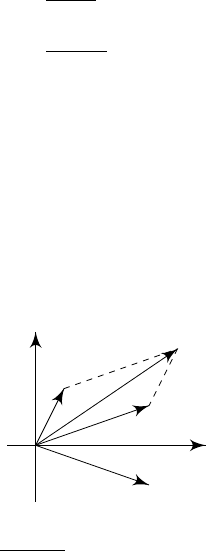1Complex numbers

IA Vectors and Matrices1.1 Basic properties
Definition
(Complex number)
.
A complex number is a number
z C
of the
form
z
=
a
+
ib
with
a, b R
, where
i
2
=
1. We write
a
=
Re
(
z
) and
b
=
Im
(
z
).
We have
z
1
± z
2
= (a
1
+ ib
1
) ± (a
2
+ ib
2
)
= (a
1
± a
2
) + i(b
1
± b
2
)
z
1
z
2
= (a
1
+ ib
1
)(a
2
+ ib
2
)
= (a
1
a
2
b
1
b
2
) + i(b
1
a
2
+ a
1
b
2
)
z
1
=
1
a + ib
=
a ib
a
2
+ b
2
Definition
(Complex conjugate)
.
The complex conjugate of
z
=
a
+
ib
is
a ib
.
It is written as ¯z or z
.
It is often helpful to visualize complex numbers in a diagram:
Definition
(Argand diagram)
.
An Argand diagram is a diagram in which a
complex number
z
=
x
+
iy
is represented by a vector
p
=
x
y
vectors corresponds to vector addition and ¯z is the reflection of z in the x-axis.
Re
Im
z
1
z
2
¯z
2
z
1
+ z
2
Definition
(Modulus and argument of complex number)
.
The modulus of
z
=
x
+
iy
is
r
=
|z|
=
p
x
2
+ y
2
. The argument is
θ
=
arg z
=
tan
1
(
y/x
). The
modulus is the length of the vector in the Argand diagram, and the argument is
the angle between z and the real axis. We have
z = r(cos θ + i sin θ)
Clearly the pair (
r, θ
) uniquely describes a complex number
z
, but each complex
number
z C
can be described by many different
θ
since
sin
(2
π
+
θ
) =
sin θ
and cos(2π + θ) = cos θ. Often we take the principle value θ (π, π].
When writing z
i
= r
i
(cos θ
i
+ i sin θ
i
), we have
z
1
z
2
= r
1
r
2
[(cos θ
1
cos θ
2
sin θ
1
sin θ
2
) + i(sin θ
1
cos θ
2
+ sin θ
2
cos θ
1
)]
= r
1
r
2
[cos(θ
1
+ θ
2
) + i sin(θ
1
+ θ
2
)]
In other words, when multiplying complex numbers, the moduli multiply and
Proposition. z¯z = a
2
+ b
2
= |z|
2
.
Proposition. z
1
= ¯z/|z|
2
.
Theorem (Triangle inequality). For all z
1
, z
2
C, we have
|z
1
+ z
2
| |z
1
| + |z
2
|.
Alternatively, we have |z
1
z
2
| ||z
1
| |z
2
||.